# Birch-Murnaghan equation of state

An extension, or rather a generalization, of the Murnaghan equation of state was presented by Albert F. Birch in 1947.  It has become known as the Birch-Murnaghan equation of state. The generalization followed from the identification that the strain energy could be approximated as a Taylor series based on the finite strain in the crystal. Common orders include first, second and third, where the first order approximation reduces to the Murnaghan equation of state.

Since finite strain is represented as: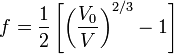$f=\frac{1}{2}\left[\left(\frac{V_0}{V}\right)^{2/3}-1\right]$

The internal energy,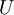$U$, for the strain is defined as a Taylor expansion: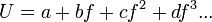$U=a+bf+cf^2+df^3...$

The pressure, then is the derivative of this equation: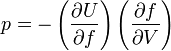$p=-\left(\frac{\partial U}{\partial f}\right)\left(\frac{\partial f}{\partial V}\right)$

The second order form is thus: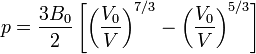$p=\frac{3B_0}{2}\left[\left(\frac{V_0}{V}\right)^{7/3}-\left(\frac{V_0}{V}\right)^{5/3}\right]$

Where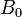$B_0$ is the isothermal (or calibration) bulk modulus. However, since this form is not dependent on the bulk modulus derivative,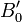$B_0'$, it is rarely used and either the first order or third order form are used. The third order shows increased accuracy over the Murnaghan equation of state and has a relatively simple analytical form (Eq. 4.42 in ):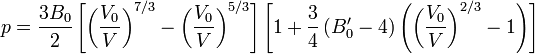$p=\frac{3B_0}{2}\left[\left(\frac{V_0}{V}\right)^{7/3}-\left(\frac{V_0}{V}\right)^{5/3}\right]\left[1+\frac{3}{4}\left(B_0'-4\right)\left(\left(\frac{V_0}{V}\right)^{2/3}-1\right)\right]$